Gaussian Copula vs Loans Loss Assessment: A Simplified and Easy-To-Use Model | OMICS International
Journal of Business & Financial Affairs

# Gaussian Copula vs Loans Loss Assessment: A Simplified and Easy-To-Use Model

Viviane Y. Naïmy*

Economics Department of Accounting, Notre Dame University, Louaize-Lebanon Faculty of Business Administration, Finance, and Economics, USA

*Corresponding Author:
Viviane Y. Naïmy
Associate Professor
Economics Department of Accounting
Finance, and Economics, Notre Dame University, USA
E-mail: [email protected]

Received June 28, 2012; Accepted July 30, 2012; PublishedAugust 02, 2012

Citation: Naimy VY (2012) Gaussian Copula vs Loans Loss Assessment: A Simplified and Easy-To-Use Model. J Bus & Fin Aff 1:105. doi:10.4172/2167-0234.1000105

Copyright: © 2012 Naimy VY . This is an open-access article distributed under the terms of the Creative Commons Attribution License, which permits unrestricted use, distribution, and reproduction in any medium, provided the original author and source are credited.

Visit for more related articles at Journal of Business & Financial Affairs

#### Abstract

The copula theory is a fundamental instrument used in modeling multivariate distributions. It defines the joint distribution via the marginal distributions together with the dependence between variables. Copulas can also model dynamic structures. This paper offers a brief description of the copulas’ statistical procedures implemented on real market data. A direct application of the Gaussian copula to the assessment of a portfolio of loans belonging to one of the banks operating in Lebanon is illustrated in order to make the implementation of the copula simple and straightforward.

#### Introduction

The concept of “copulas1” was named by Sklar . In statistics, the word copula describes the function that “joins” one dimensional distribution functions to form multivariate ones, and may serve to characterize several dependence concepts. It was initiated in the context of probabilistic metric spaces. Copulas function became a popular multivariate modeling tool in financial applications since 1999 . They are used for asset allocation, credit scoring, default risk modeling, derivative pricing, and risk management [3,4]. They became more and more popular because as proven, the returns of financial assets are non-Gaussian and show nonlinearities; therefore, copulas became an imperative modeling device in a non-Gaussian world. They implement algorithms to simulate asset return distributions in a realistic way by modeling a multivariate dependence structure separately from the marginal distributions.

For multivariate distributions, the univariate margins and the dependence structure can be separated and the latter may be represented by a copula. The copula of a multivariate distribution whose marginals are all uniform over (0,1). Sklar’s Theorem states that a d-dimensional cumulative distribution function F evaluated at point X = (x1, …, xd) can be represented as

F(X) = C (F1(x1), . . . , Fd(xd))

Where C is the copula function and Fi, i = 1, ..d, are the margins. In most cases the latter function is uniquely defined by (1.1).

1The word copula, resp. copulare, is a latin noun, resp. verb, that means “bond”, resp. “to connect” or “to join”. Th e term copula is used in grammar and logic to describe that part of a proposition which connects the subject and predicate.

If F1, F2 are continuous, then C is unique; otherwise, C is uniquely determined on Ran (F1) x Ran (F2). Conversely, if C is a copula and F1, F2 are distribution functions, then the function F defined in (1) is a joint distribution function with margins F1 and F2. For the proof demonstration, please refer to Nelsen . It is the converse of the Sklar’s theorem that is mostly used for modeling multivariate distributions in finance. Any group of n univariate distributions of any type can be linked with any copula while still obtaining a valid multivariate distribution.

Using copulas is attractive to practitioners because even under increasing transformations of the margins, copulas remain invariant. This requires the completion of two steps: modeling each univariate marginal distribution then specifying a copula that recapitulates all the dependencies between margins.

In this paper our aim is to simplify the implementation of copulas for the Lebanese banks since none of which is using copulas for risk assessment. Section 1 introduces the model that generates the copula through the Archimedian and elliptical copulas. Section 2 illustrates the use of the Gaussian copula in the assessment of a portfolio of loans belonging to one of the banks operating in Lebanon. Section 3 draws some concluding remarks.

#### Definition and Families of Copulas

The quantification of dependence in finance has led to the development of copulas. Copulas are now witnessing increasing interest in many areas of risk analysis. The rank order correlation2 used by most Monte Carlo simulation tools is certainly a meaningful measure of dependence but is still limited in the patterns it can produce.

As described in the introduction, copulas offer an efficient flexible procedure for combining marginal distributions into multivariate distributions and are able to deduce the real correlation pattern. The purpose of this paper is not to go through the explanations and proof of the mathematics related to copulas (that can be found in any related book), we will rather simplify the concept and depict what is just needed to run an analysis using the copula.

2The rank order correlation coefficient uses the ranking of the data, i.e. what position (rank) the data point takes in an ordered list from the minimum to maximum values, rather than the actual data values themselves. It is therefore independent of the distribution shapes of the data sets and allows the integrity of the input distributions to be maintained. Spearman’s rho is calculated as: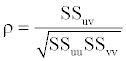Where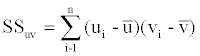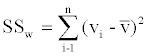and where ui, vi are the ranks of the ith observation in samples 1 and 2 respectively. The correlation coefficient is symmetric i.e., only the difference between ranks is important and not whether distribution 1 is being correlated with distribution 2 or the other way round.

To this end, in what follows, we use the needed formulae for a bivariate copula for simplicity purpose. Related graphs of bivariate copulas will be illustrated. Multivariate copulas can be extended based on the bivariate concept.

Because the copula of a multivariate distribution describes its dependence structure, we can use measures of dependence such as Kendall’s3 tau and Spearman’s4 rho, as well as the coefficient of tail dependence, which are copula-based. The relationship between Kendall’s tau t of two variables X and Y and the copula C(u,v) of the bivariate distribution function of X and Y gives a tool for fitting a copula to a set of data. This consists in determining Kendall’s tau then applying a transformation in order to get the appropriate parameters for the fitted copula.

3Kendall’s tau and Spearman’s rho provide the best alternatives to the linear correlation coefficient as a measure of dependence for nonelliptical distributions, for which the linear correlation coefficient is inappropriate and often misleading. For more details we refer to Kendall and Stuart , Kruskal , Lehmann  and Capéraà and Genest .

Kendall’s tau for a two observed sets of variables is given by: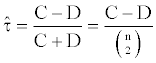Where C is the number of concordant pairs and D the number of discordant pairs. This can also be written as: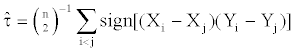This is used to measure the degree of correspondence between two variables (paired observations): Perfect correspondence between the two variables means that the coefficient has a value of 1

Perfect disagreement between the two rankings means that the coefficient has a value of -1.

For all other arrangements, the value lies between -1 and 1, 0 meaning the variables are completely independent.

Therefore, Kendall’s tau for (X, Y)T is simply the probability of concordance minus the probability of discordance.

4Kendall’s tau and Spearman’s rho are both carried out on the ranks of the data as explained and defined in note 2. That is, for each variable separately the values are put in order and numbered.

The copula families

The most frequently used copula families are the elliptical and Archimedean copulas. Table 1summarizes and compares the two families.

Elliptical Copulas Archimedean Copulas
1. Definition Elliptical copulas are the copulas of elliptically contoured (or elliptical) distributions. The most famous elliptical distributions are the multivariate:
1. Normal5(Gaussian copula) and
2. Student-t6distributions.
Archimedean copulas are easily constructed and enjoy attractive properties. The most commonly used distributions are:
1. Clayton7
2. Frank8
3. Gumbel9
The relationship between thelinear correlation coefficient ρ and Kendall’s tau t for all the elliptical copulas’ families is given by: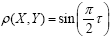The general relationship
between Kendall's tau t and the generator of an Archimedean copula φa(t)for a bivariate data set is given by: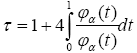2. Advantages Different levels of correlation between the
marginals can be easily determined.
Easy to be deduced.
3. Disadvantages Absence of closed form expressions,
The definition doesn't extend
to a multivariate data set of n variables because there will be multiple values of tau, one for each pairing10.
Table 1: Comparison between the most frequently used Copulas.

5The normal copula is given by: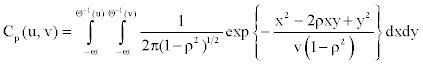6The Student-t copula is an elliptical copula defined as: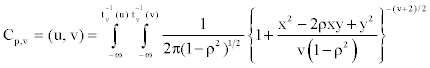Where v (the number of degrees of freedom) and ρ (linear correlation coefficient) are the parameters of the copula. When v is large (greater than 30) the copula converges to the Normal copula just as the Student distribution converges to the Normal. In the opposite case, the behavior of the copulas is different: the t-copula has more points in the tails than the Gaussian one and a star like shape. A Student-t copula with n = 1 is sometimes called a Cauchy copula.

7It is an asymmetric copula, exhibiting greater dependence in the negative tail than in the positive. This copula is written as: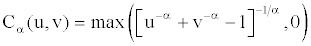Its generator is: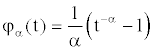Where: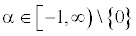The relationship between Kendall’s tau τ and the Clayton copula parameter α is given by: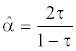8It is a symmetric copula given by: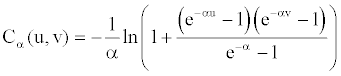Its generator is: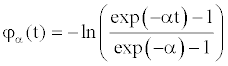Where: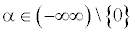The relationship between Kendall’s tau τ and the Frank copula parameter α is given by: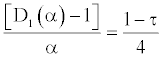Where: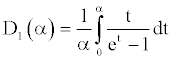9The Gumbel-Hougard copula is asymmetric, exhibiting greater dependence in the positive tail than in the negative. It is given by: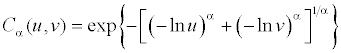Its generator is: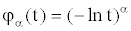Where: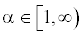The relationship between Kendall’s tau τ and the Gumbel copula parameter α is given by: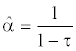10This constraint can be solved by calculating tau for each pair and then using the average (Figure 1).

#### Applications

The purpose of this section is to illustrate a direct example on how to implement a one factor model of the Gaussian copula (normal copula) on a portfolio of loans belonging to a Lebanese bank operating in Lebanon .

The portfolio is constituted of 30 different companies. We define Ti(1 ≤ i ≤ N) as the date upon which the company will default. We consider that all the studied companies in the portfolio can eventually default  however; this cannot happen except over long periods sometimes exceeding 50 years or more.

The model

Qi is the distribution of Ti. In order to define a correlation structure among the Ti based on a one factor model of a Gaussian copula, we have transformed each variable, percentile per percentile, into a Ui variable, following a standardized bivariate distribution. We followed the below factorial model (one factor model) for the correlation structure

Ui = aiF + √(1-a2iZi)

Where F and Zi follow a normal distribution N(0,1). The link between Uiand Ti implies:

Prob(Ui<U) = Prob(Ti<T)

Where U = N-1 [Qi (T)]

According to equation (2), the probability of Ui< U conditional to the value of F is:

Prob(Ui<U/F) = N[U-aiF)/(√(1-a2iZi)]

Equation (4) can be written for Prob(Ti<T):

Prob(Ti<T/F) = N[(N-1 [Qi(T)]-aiF)/(√(1-a2i)]

In order to simplify the reasoning process, we can assume that the time distribution of default Qi is the same for each i and equals Q. We also assume for simplicity purpose that the copula correlation between each pair of companies is the same and equals ρ. In this case, for each pair of companies i and j, the correlation is aiaj, which means that for each i, ai = √ρ. Therefore we can rewrite equation (5) as follow:

Prob(Ti<T/F) = N[(N-1 [Q (T)]- √ρ F)/(√(1-ρ)]

For a portfolio constituted of a huge number of loans, this equation offers a valid approximation of the proportion of companies that can default on date T. We name this proportion the default rate. When F decreases, the default rate increases. Therefore what is the cap level that this rate can reach? Since F follows a standardized bivariate distribution, the probability for F to have a value less than N-1(Y) is Y. Therefore, there is a probability Y for the default rate to be greater than:

N[(N-1 [Q (T)]- √ρ N-1(Y))/(√(1-ρ)]

We note that the default rate V(T,X) will not be exceeded with a probability of X. In other words, we are sure up to X% that the default rate V(T,X) will not be exceeded. The value V(T,X) is measured by substituting 1-X to Y:

V(T,X)= N[(N-1 [Q (T)]+ √ρ N-1(X))/(√(1-ρ)]

This output has been first developed by Vasicek .

If we assume that the total value of the loan in USD is L and the recovery rate in case of default is R. The VaR of a loan over a horizon period of T and a confidence level of X is:

VaR(T,X) = L × R × V(T,X)

The sample

We were able to analyze the loan portfolio of a Lebanese bank operating in Lebanon. For discretion purpose we will omit the name of the bank however, the below tables 2, 3 and figures 2,3 are enough to give an idea about the portfolio details and the financial performance of this bank. The main purpose of this section is to directly implement the Normal copula model in order to measure the expected loss on a portfolio of loan.

IFRS11
1133/31/13 1131/31/13 1112/31/13 1112/31/13
Assets Quality
Loan Loss Res. / Gross Loans 35.4 45.3 45.3 45.3
Loan Loss Prov. / Net Int. Rev .0503 3533 35.. .5.4
Loan Loss Res / Impaired Loans 50.5.. 5..54. 54.5.. .3.5.
Impaired Loans / Gross Loans 555. .534 .534 5554
NCO / Average Gross Loans 05.- 055.- 0504- n.a5
NCO / Net Inc Bef. Ln. Loss 550.- 35.3- 053.- n.a5
Impaired Loans / Equity .534 4533 35.. .5.3
Equity (Bil LBP) 2,484.800 2,456.400 1,952.800 1,618.409
Net Income (Bil LBP) 270.900 267.800 219.400 183.915
Capital
Total Capital Ratio 13.61 14.70 15.31 24.10
Equity / Total Assets 9.93 10.66 9.54 9.56
Capital Funds / Liabilities 12.56 13.60 12.37 12.75
Operations
Net Interest Margin 2.12 2.29 2.42 2.76
Return on Average Assets 1.13 1.23 1.17 1.17
Return on Average Equity 10.97 12.15 12.29 13.28
Cost to Income Ratio 43.38 45.53 46.28 47.34
Liquidity
Net Loans / Total Assets 24.14 24.67 23.55 24.85
Net Loans / Customer & ST 28.80 29.14 28.03 29.72
Liquid Assets / Cust & ST 28.66 24.26 34.20 33.64

Table 2: Selected Consolidated Statements.

Description Variables
N 30
Type of Companies SMEs
Type of loans Commercial
Average Term of the loans 78 months
Average loan’s size USD 1.8 millions
Interest rate type Floating
Average interest rate 9.75%

Table 3: Description of the Portfolio.

11International Financial Reporting Standards

#### Results

The total amount of loan on which we applied the model is USD 56.4 millions. Based on our observation we have been able to determine a default probability over a horizon period of one year of 2%. The recovered amount in case of default was quantified by the bank at a level of 72%. Using the Vose Software12 we deduced a copula correlation rate of 0.1.

Based on the above and according to equation (7):

V(1, 0.999) = N[(N-1 [0.02]+ √0.1 N-1(0.999))/(√(1-0.1)] = 0.128

In other words, the bank is 99% confident that the default rate cannot exceed 12.8%. Therefore the Value at Risk, VaR, or the losses cannot exceed:

0.128 × 56.4 × (1-0.72) = USD 2, 021,376

#### Concluding Remarks

This work showed how the Gaussian copulas theory can be very powerful tool in estimating the expected losses on a portfolio of loans.

Risk managers often estimate the marginal distribution between each of the variables and face the obligation to set hypothesis on the structure of the variables’ correlation. When the marginal distributions are normal, it is natural to consider that these variables follow multivariate normal distributions. In the opposite cases, copulas are therefore used. Variables are transformed into normal variables bases on a percentile per percentile adjustment process. The correlation is then indirectly defined among the transformed variables. When many variables are studied, a factorial model is used by analysts. This approach allows reducing the number of correlation to be calculated. The correlations between variables are described by the correlations of variables toward the common factor. The default correlation between many firms can be modeled based on a Gaussian copula factorial model.

Despite all the benefits of copula models, specifying a copula that recapitulates all the dependencies between margins suffers from some statistical pitfalls especially for most of multivariate financial series. This is due to temporal dependencies such as serial autocorrelation, or time varying heteroskedasticity, etc. Further research must be done in order to broaden the scope of implementations to higher dimensions while considering the limitations of copulas in general.

#### References

Select your language of interest to view the total content in your interested language

### Article Usage

• Total views: 13651
• [From(publication date):
September-2012 - Apr 03, 2020]
• Breakdown by view type
• HTML page views : 9811Can't read the image? click here to refresh